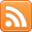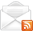# ASP.NET,C#.NET,VB.NET,JQuery,JavaScript,Gridview

aspdotnet-suresh offers C#.net articles and tutorials,csharp dot net,asp.net articles and tutorials,VB.NET Articles,Gridview articles,code examples of asp.net 2.0 /3.5,AJAX,SQL Server Articles,examples of .net technologies

### SQL Server Arithmetic Overflow Error Converting Numeric (Money) to Data Type Numeric

Aug 4, 2015
Introduction:

Here I will explain how to fix / solve problem of “
Arithmetic overflow error converting numeric to data type numeric.” in sql server. Actually this problem occured whenever we are converting decimal or money value to numeric datatype with limited size in sql server. To fix this error we need to increase numeric datatype size limit then automatically this problem fix in sql server.
Description:
In previous articles I explained SQL Server single procedure to insert update delete, SQL Server take database backup, SQL Server restore database from .bak / .mdf file, Primary key constraint in sql server, foreign key constraint in sql server, cursor example in sql server and many articles relating to SQL server. Now I will explain how to fix problem of Arithmetic overflow error converting numeric to data type numeric.” in sql server.

Now we will see how we will get this error and fix for this with one example for that write the following query and execute it

 DECLARE @decval DECIMAL SET @decval = 503.12 DECLARE @numval NUMERIC(3,2) SET @numval = CONVERT(NUMERIC,@decval) print @numval
When we run above query we will get output like as shown below

Output

We got this problem because of following lines of query

 SET @decval = 503.12 DECLARE @numval NUMERIC(3,2) SET @numval = CONVERT(NUMERIC,@decval)
Here NUMERIC(3,2) means overall it will allow only 3 digits within that 2 are decimal values but here @decval = 503.12 so overall 5 digits including decimal value but in @numval we are allowing only 3 digits including decimal value that’s the reason it’s throwing error.

To fix this problem we need to increase number of digits size in numeric datatype. In NUMERIC(a,b) we need to increase “a” value.

Now we will make modification in above query and write it like as shown below

 DECLARE @decval DECIMAL SET @decval = 503.12 DECLARE @numval NUMERIC(5,2) SET @numval = CONVERT(NUMERIC,@decval) print @numval
If we execute above query we will get output like as shown below

Output

I hope it helps you to fix your problem….
 If you enjoyed this post, please support the blog below. It's FREE! Get the latest Asp.net, C#.net, VB.NET, jQuery, Plugins & Code Snippets for FREE by subscribing to our Facebook, Twitter, RSS feed, or by email.Subscribe by RSSSubscribe by Email